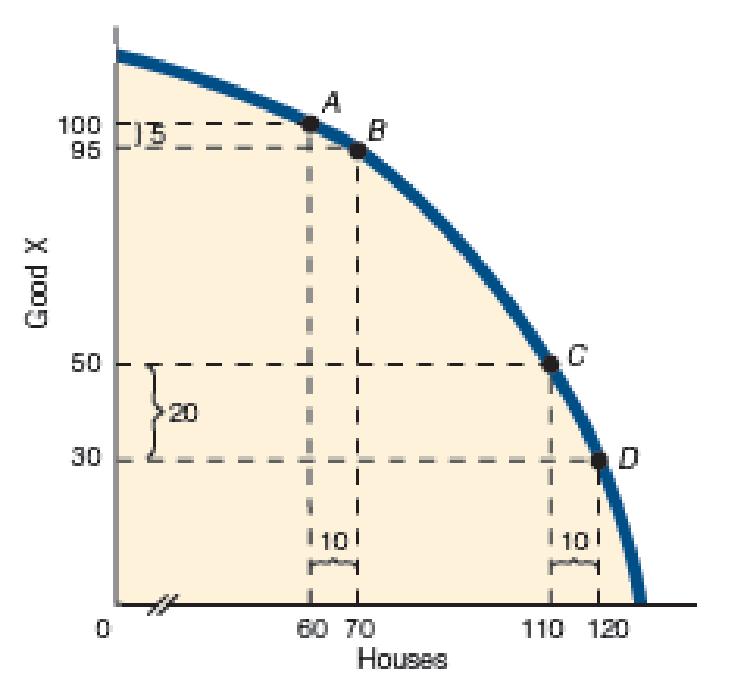Chapter 2, Problem 4QP

Chapter
Section
Textbook Problem

Look back at Exhibit 4 and notice that the slope between points A and B is relatively flatter than it is between points C and D. What does the slope of a curve between two points have to do with the opportunity cost of producing additional units of a good?To determine

Calculation of slope.

Explanation

Figure 1 shows the PPF curve of good X and houses.

In figure 1, vertical axis measures the good X and horizontal axis measures houses. The concave curve is the PPF (production possibility frontier).

The general formula for calculating slope is given below.

Slope=Cost of good Xpresent Costof good XpreviousQuantity of housespresent Quantity housesprevious        (1)

Substitute the respective values in equation (1) to calculation the slope of AB

Still sussing out bartleby?

Check out a sample textbook solution.

See a sample solution

The Solution to Your Study Problems

Bartleby provides explanations to thousands of textbook problems written by our experts, many with advanced degrees!

Get Started

Explain why an economys income must equal its expenditure.

Brief Principles of Macroeconomics (MindTap Course List)

Why will global sourcing increase?

Purchasing and Supply Chain Management

What does cross-referencing mean in the posting process?

College Accounting (Book Only): A Career Approach

WACC The Patrick Companys year-end balance sheet is shown below. Its cost of common equity is 16%, its before-t...

Fundamentals of Financial Management, Concise Edition (with Thomson ONE - Business School Edition, 1 term (6 months) Printed Access Card) (MindTap Course List)

Explain the following statement: Our tax rates are progressive.

Fundamentals of Financial Management, Concise Edition (MindTap Course List)

Define discounting and compounding.

Intermediate Accounting: Reporting And Analysis

What is a partial dependency?

Pkg Acc Infor Systems MS VISIO CD